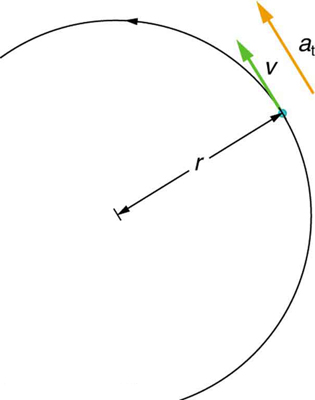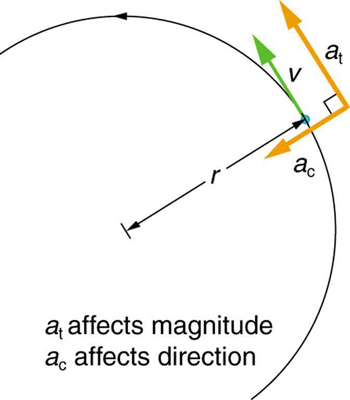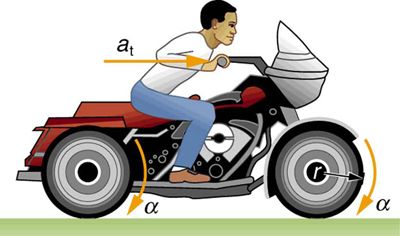Chapter 10 Rotational Motion and Angular Momentum

# 67 10.1 Angular Acceleration

### Summary

• Describe uniform circular motion.
• Explain non-uniform circular motion.
• Calculate angular acceleration of an object.
• Observe the link between linear and angular acceleration.

Chapter 6 Uniform Circular Motion and Gravitation discussed only uniform circular motion, which is motion in a circle at constant speed and, hence, constant angular velocity. Recall that angular velocity$\boldsymbol{\omega}$was defined as the time rate of change of angle$\boldsymbol{\theta}:$

$\boldsymbol{\omega\:=}$$\boldsymbol{\frac{\Delta\theta}{\Delta{t}}},$

where$\boldsymbol{\theta}$is the angle of rotation as seen in Figure 1. The relationship between angular velocity$\boldsymbol{\omega}$and linear velocity$\boldsymbol{v}$was also defined in Chapter 6.1 Rotation Angle and Angular Velocity as

$\boldsymbol{v=r\omega}$

or

$\boldsymbol{\omega\:=}$$\boldsymbol{\frac{v}{r}},$

where$\boldsymbol{r}$is the radius of curvature, also seen in Figure 1. According to the sign convention, the counter clockwise direction is considered as positive direction and clockwise direction as negative

Angular velocity is not constant when a skater pulls in her arms, when a child starts up a merry-go-round from rest, or when a computer’s hard disk slows to a halt when switched off. In all these cases, there is an angular acceleration, in which$\boldsymbol{\omega}$changes. The faster the change occurs, the greater the angular acceleration. Angular acceleration$\boldsymbol{\alpha}$is defined as the rate of change of angular velocity. In equation form, angular acceleration is expressed as follows:

$\boldsymbol{\alpha\:=}$$\boldsymbol{\frac{\Delta\omega}{\Delta{t}}},$

where$\boldsymbol{\Delta\omega}$is the change in angular velocity and$\boldsymbol{\Delta{t}}$is the change in time. The units of angular acceleration are$\textbf{(rad/s)/s},$or$\boldsymbol{\textbf{rad/s}^2}.$If$\boldsymbol{\omega}$increases, then$\boldsymbol{\alpha}$is positive. If$\boldsymbol{\omega}$decreases, then$\boldsymbol{\alpha}$is negative.

### Example 1: Calculating the Angular Acceleration and Deceleration of a Bike Wheel

Suppose a teenager puts her bicycle on its back and starts the rear wheel spinning from rest to a final angular velocity of 250 rpm in 5.00 s. (a) Calculate the angular acceleration in$\boldsymbol{\textbf{rad/s}^2}.$(b) If she now slams on the brakes, causing an angular acceleration of$\boldsymbol{-87.3\textbf{ rad/s}^2},$how long does it take the wheel to stop?

Strategy for (a)

The angular acceleration can be found directly from its definition in$\boldsymbol{\alpha=\frac{\Delta\omega}{\Delta{t}}}$because the final angular velocity and time are given. We see that$\boldsymbol{\Delta\omega}$is 250 rpm and$\boldsymbol{\Delta{t}}$is 5.00 s.

Solution for (a)

Entering known information into the definition of angular acceleration, we get

$\begin{array}{lcl} \boldsymbol{\alpha} & \boldsymbol{=} & \boldsymbol{\frac{\Delta\omega}{\Delta{t}}} \\ {} & \boldsymbol{=} & \boldsymbol{\frac{250\textbf{ rpm}}{5.00\textbf{ s.}}} \end{array}$

Because$\boldsymbol{\Delta\omega}$is in revolutions per minute (rpm) and we want the standard units of$\boldsymbol{\textbf{rad/s}^2}$for angular acceleration, we need to convert$\boldsymbol{\Delta\omega}$from rpm to rad/s:

$\begin{array}{lcl} \boldsymbol{\Delta\omega} & \boldsymbol{=} & \boldsymbol{250\frac{\textbf{rev}}{\textbf{min}}\cdotp\frac{2\pi\textbf{ rad}}{\textbf{rev}}\cdotp\frac{1\textbf{ min}}{60\textbf{ sec}}} \\ {} & \boldsymbol{=} & \boldsymbol{26.2\textbf{ rads.}} \end{array}$

Entering this quantity into the expression for$\boldsymbol{\alpha},$we get

$\begin{array}{lcl} \boldsymbol{\alpha} & \boldsymbol{=} & \boldsymbol{\frac{\Delta\omega}{\Delta{t}}} \\ {} & \boldsymbol{=} & \boldsymbol{\frac{26.2\textbf{ rad/s}}{5.00\textbf{ s}}} \\ {} & \boldsymbol{=} & \boldsymbol{5.24\textbf{ rad/s.}^2} \end{array}$

Strategy for (b)

In this part, we know the angular acceleration and the initial angular velocity. We can find the stoppage time by using the definition of angular acceleration and solving for$\boldsymbol{\Delta{t}},$yielding

$\boldsymbol{\Delta{t}\:=}$$\boldsymbol{\frac{\Delta\omega}{\alpha}}.$

Solution for (b)

Here the angular velocity decreases from$\boldsymbol{26.2\textbf{ rad/s}}$(250 rpm) to zero, so that$\boldsymbol{\Delta\omega}$is$\boldsymbol{-26.2\textbf{ rad/s}},$and$\boldsymbol{\alpha}$is given to be$\boldsymbol{-87.3\textbf{ rad/s}^2}.$Thus,

$\begin{array}{lcl} \boldsymbol{\Delta{t}} & \boldsymbol{=} & \boldsymbol{\frac{-26.2\textbf{ rad/s}}{-87.3\textbf{ rad/s}^2}} \\ {} & \boldsymbol{=} & \boldsymbol{0.300\textbf{ s.}} \end{array}$

Discussion

Note that the angular acceleration as the girl spins the wheel is small and positive; it takes 5 s to produce an appreciable angular velocity. When she hits the brake, the angular acceleration is large and negative. The angular velocity quickly goes to zero. In both cases, the relationships are analogous to what happens with linear motion. For example, there is a large deceleration when you crash into a brick wall—the velocity change is large in a short time interval.

If the bicycle in the preceding example had been on its wheels instead of upside-down, it would first have accelerated along the ground and then come to a stop. This connection between circular motion and linear motion needs to be explored. For example, it would be useful to know how linear and angular acceleration are related. In circular motion, linear acceleration is tangent to the circle at the point of interest, as seen in Figure 2. Thus, linear acceleration is called tangential acceleration$\boldsymbol{a_{\textbf{t}}}.$Figure 2. In circular motion, linear acceleration a, occurs as the magnitude of the velocity changes: a is tangent to the motion. In the context of circular motion, linear acceleration is also called tangential acceleration at.

Linear or tangential acceleration refers to changes in the magnitude of velocity but not its direction. We know from Chapter 6 Uniform Circular Motion and Gravitation that in circular motion centripetal acceleration,$\boldsymbol{a_{\textbf{c}}},$refers to changes in the direction of the velocity but not its magnitude. An object undergoing circular motion experiences centripetal acceleration, as seen in Figure 3. Thus,$\boldsymbol{a_{\textbf{t}}}$and$\boldsymbol{a_{\textbf{c}}}$are perpendicular and independent of one another. Tangential acceleration$\boldsymbol{a_{\textbf{t}}}$is directly related to the angular acceleration$\boldsymbol{\alpha}$and is linked to an increase or decrease in the velocity, but not its direction.Figure 3. Centripetal acceleration ac occurs as the direction of velocity changes; it is perpendicular to the circular motion. Centripetal and tangential acceleration are thus perpendicular to each other.

Now we can find the exact relationship between linear acceleration$\boldsymbol{a_{\textbf{t}}}$and angular acceleration$\boldsymbol{\alpha}.$Because linear acceleration is proportional to a change in the magnitude of the velocity, it is defined (as it was in Chapter 2 One-Dimensional Kinematics) to be

$\boldsymbol{a_{\textbf{t}}\:=}$$\boldsymbol{\frac{\Delta{v}}{\Delta{t}}.}$

For circular motion, note that$\boldsymbol{v=r\omega},$so that

$\boldsymbol{a_{\textbf{t}}\:=}$$\boldsymbol{\frac{\Delta(r\omega)}{\Delta{t}}.}$

The radius$\boldsymbol{r}$is constant for circular motion, and so$\boldsymbol{\Delta(r\omega)=r(\Delta\omega)}.$Thus,

$\boldsymbol{a_{\textbf{t}}=r}$$\boldsymbol{\frac{\Delta\omega}{\Delta{t}}.}$

By definition,$\boldsymbol{\alpha=\frac{\Delta\omega}{\Delta{t}}}.$Thus,

$\boldsymbol{a_{\textbf{t}}=r\alpha},$

or

$\boldsymbol{\alpha\:=}$$\boldsymbol{\frac{a_{\textbf{t}}}{r}.}$

These equations mean that linear acceleration and angular acceleration are directly proportional. The greater the angular acceleration is, the larger the linear (tangential) acceleration is, and vice versa. For example, the greater the angular acceleration of a car’s drive wheels, the greater the acceleration of the car. The radius also matters. For example, the smaller a wheel, the smaller its linear acceleration for a given angular acceleration$\boldsymbol{\alpha}.$

### Example 2: Calculating the Angular Acceleration of a Motorcycle Wheel

A powerful motorcycle can accelerate from 0 to 30.0 m/s (about 108 km/h) in 4.20 s. What is the angular acceleration of its 0.320-m-radius wheels? (See Figure 4.)Figure 4. The linear acceleration of a motorcycle is accompanied by an angular acceleration of its wheels.

Strategy

We are given information about the linear velocities of the motorcycle. Thus, we can find its linear acceleration$\boldsymbol{a_{\textbf{t}}}.$Then, the expression$\boldsymbol{\alpha=\frac{a_{\textbf{t}}}{r}}$ can be used to find the angular acceleration.

Solution

The linear acceleration is

$\begin{array}{lcl} \boldsymbol{a_{\textbf{t}}} & \boldsymbol{=} & \boldsymbol{\frac{\Delta{v}}{\Delta{t}}} \\ {} & \boldsymbol{=} & \boldsymbol{\frac{30.0\textbf{ m/s}}{4.20\textbf{ s}}} \\ {} & \boldsymbol{=} & \boldsymbol{7.14\textbf{ m/s}^2.} \end{array}$

We also know the radius of the wheels. Entering the values for$\boldsymbol{a_{\textbf{t}}}$and$\boldsymbol{r}$into$\boldsymbol{\alpha=\frac{a_{\textbf{t}}}{r}},$we get

$\begin{array}{lcl} \boldsymbol{\alpha} & \boldsymbol{=} & \boldsymbol{\frac{a_{\textbf{t}}}{r}} \\ {} & \boldsymbol{=} & \boldsymbol{\frac{7.14\textbf{ m/s}}{20.320\textbf{ m}}} \\ {} & \boldsymbol{=} & \boldsymbol{22.3\textbf{ rad/s}^2.} \end{array}$

Discussion

Units of radians are dimensionless and appear in any relationship between angular and linear quantities.

So far, we have defined three rotational quantities— $\boldsymbol{\theta,\:\omega},$and$\boldsymbol{\alpha}.$These quantities are analogous to the translational quantities$\boldsymbol{x},\:\boldsymbol{v},$and$\boldsymbol{a}.$Table 1 displays rotational quantities, the analogous translational quantities, and the relationships between them.

Rotational Translational Relationship
$\boldsymbol{\theta}$ $\boldsymbol{x}$ $\boldsymbol{\theta=\frac{x}{r}}$
$\boldsymbol{\omega}$ $\boldsymbol{v}$ $\boldsymbol{\omega=\frac{v}{r}}$
$\boldsymbol{\alpha}$ $\boldsymbol{a}$ $\boldsymbol{\alpha=\frac{a_{\textbf{t}}}{r}}$
Table 1. Rotational and Translational Quantities.

### MAKING CONNECTIONS: TAKE-HOME EXPERIMENT

Sit down with your feet on the ground on a chair that rotates. Lift one of your legs such that it is unbent (straightened out). Using the other leg, begin to rotate yourself by pushing on the ground. Stop using your leg to push the ground but allow the chair to rotate. From the origin where you began, sketch the angle, angular velocity, and angular acceleration of your leg as a function of time in the form of three separate graphs. Estimate the magnitudes of these quantities.

1: Angular acceleration is a vector, having both magnitude and direction. How do we denote its magnitude and direction? Illustrate with an example.

Join the ladybug in an exploration of rotational motion. Rotate the merry-go-round to change its angle, or choose a constant angular velocity or angular acceleration. Explore how circular motion relates to the bug’s x,y position, velocity, and acceleration using vectors or graphs.

# Section Summary

• Uniform circular motion is the motion with a constant angular velocity$\boldsymbol{\omega=\frac{\Delta\theta}{\Delta{t}}}.$
• In non-uniform circular motion, the velocity changes with time and the rate of change of angular velocity (i.e. angular acceleration) is$\boldsymbol{\alpha=\frac{\Delta\omega}{\Delta{t}}}.$
• Linear or tangential acceleration refers to changes in the magnitude of velocity but not its direction, given as$\boldsymbol{a_{\textbf{t}}=\frac{\Delta{v}}{\Delta{t}}}.$
• For circular motion, note that$\boldsymbol{v=r\omega},$so that
$\boldsymbol{a_{\textbf{t}}\:=}$$\boldsymbol{\frac{\Delta(r\omega)}{\Delta{t}}}.$
• The radius r is constant for circular motion, and so$\boldsymbol{\Delta(r\omega)=r\Delta\omega}.$Thus,
$\boldsymbol{a_{\textbf{t}}=r}$$\boldsymbol{\frac{\Delta\omega}{\Delta{t}}}.$
• By definition,$\boldsymbol{\Delta\omega/\Delta{t}=\alpha}.$Thus,
$\boldsymbol{a_{\textbf{t}}=r\alpha}$

or

$\boldsymbol{\alpha=}$$\boldsymbol{\frac{a_{\textbf{t}}}{r}}.$

### Conceptual Questions

1: Analogies exist between rotational and translational physical quantities. Identify the rotational term analogous to each of the following: acceleration, force, mass, work, translational kinetic energy, linear momentum, impulse.

2: Explain why centripetal acceleration changes the direction of velocity in circular motion but not its magnitude.

3: In circular motion, a tangential acceleration can change the magnitude of the velocity but not its direction. Explain your answer.

4: Suppose a piece of food is on the edge of a rotating microwave oven plate. Does it experience nonzero tangential acceleration, centripetal acceleration, or both when: (a) The plate starts to spin? (b) The plate rotates at constant angular velocity? (c) The plate slows to a halt?

### Problems & Exercises

1: At its peak, a tornado is 60.0 m in diameter and carries 500 km/h winds. What is its angular velocity in revolutions per second?

2: Integrated Concepts

An ultracentrifuge accelerates from rest to 100,000 rpm in 2.00 min. (a) What is its angular acceleration in$\boldsymbol{\textbf{rad/s}^2}?$(b) What is the tangential acceleration of a point 9.50 cm from the axis of rotation? (c) What is the radial acceleration in$\boldsymbol{\textbf{m/s}^2}$and multiples of$\boldsymbol{g}$of this point at full rpm?

3: Integrated Concepts

You have a grindstone (a disk) that is 90.0 kg, has a 0.340-m radius, and is turning at 90.0 rpm, and you press a steel axe against it with a radial force of 20.0 N. (a) Assuming the kinetic coefficient of friction between steel and stone is 0.20, calculate the angular acceleration of the grindstone. (b) How many turns will the stone make before coming to rest?

4: Unreasonable Results

You are told that a basketball player spins the ball with an angular acceleration of$\boldsymbol{100\textbf{ rad/s}^2}.$(a) What is the ball’s final angular velocity if the ball starts from rest and the acceleration lasts 2.00 s? (b) What is unreasonable about the result? (c) Which premises are unreasonable or inconsistent?

## Glossary

angular acceleration
the rate of change of angular velocity with time
change in angular velocity
the difference between final and initial values of angular velocity
tangential acceleration
the acceleration in a direction tangent to the circle at the point of interest in circular motion

### Solutions

1: The magnitude of angular acceleration is$\boldsymbol{\alpha}$and its most common units are$\boldsymbol{\textbf{rad/s}^2}.$The direction of angular acceleration along a fixed axis is denoted by a + or a – sign, just as the direction of linear acceleration in one dimension is denoted by a + or a – sign. For example, consider a gymnast doing a forward flip. Her angular momentum would be parallel to the mat and to her left. The magnitude of her angular acceleration would be proportional to her angular velocity (spin rate) and her moment of inertia about her spin axis.

Problems & Exercises

1:

$\boldsymbol{\omega=0.737\textbf{ rev/s}}$

3:

(a)$\boldsymbol{-0.26\textbf{ rad/s}^2}$

(b)$\boldsymbol{27\textbf{ rev}}$